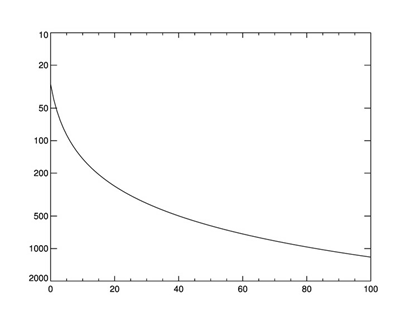# Labelling Logarithmic Axes

QUESTION: I am plotting pressure on the Y axis and it varies from about 1200 mbar at the surface to about 30 mbar at the top of the layer I am plotting. Since I am using a logarithmic axis scale, the major tick marks are at 1000 and 100 and labelled this way on the plot. But I would desperately like some of the minor tick marks to also be labelled. For example, I would like labels at 200 and 500 mbar. But no amount of fooling around with the number of major or minor tick marks seems to help. Is there a way to force IDL to label these “minor” tick marks?ANSWER: This is one of the perennial top-ten requests for new IDL features and I am surprised it has taken me this long to write an article about it. Thanks for asking!

My personal opinion is that IDL ought to be able to do this on its own, but it doesn't, so there is no use worrying about it. Here is how I do it, using the very useful routine LogLevels, written by Martin Schultz.

The trick is simply to match the number of major tick marks (set with YTICKS) with the number of values being returned from LogLevels The number of YTICKS is always one less than the number of values returned from LogLevels. In this case, I wish to plot the data, which has a range of 30 to 1200, using an axis range of 10 to 2000, and I wish to label the minor tick marks at 20, 50, 200, 500, etc. Because this is pressure data, I will also plot the highest pressure at the bottom of the Y axis. I use the useful Coyote Library routine cgScaleVector to prepare the data.

```   data = cgScaleVector(Findgen(101), 30, 1200)
ticks = LogLevels([10,2000])
nticks = N_Elements(ticks)
cgPlot, data, /YLOG, YRANGE=[2000,10], YTICKS=nticks-1, \$
YTICKV=Reverse(ticks)
```

Of course, if you don't reverse the direction of the Y axis, there is no need to use the REVERSE function with the YTICKV keyword in the code above. (But, I'm sure you knew that!)The minor tick marks are labelled on this logarithmic axis.Web Coyote's Guide to IDL Programming